Скачать презентацию Jan 29, 2016 11 Probability Theory

seminar_1_probability.ppt

• Размер: 575 Кб
• Количество слайдов: 52

## Описание презентации Jan 29, 2016 11 Probability Theory по слайдам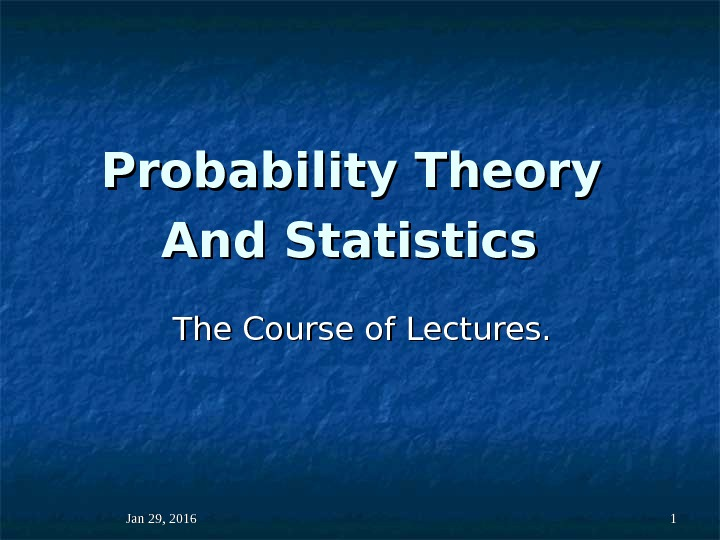Jan 29, 2016 11 Probability Theory And Statistics The Course of Lectures.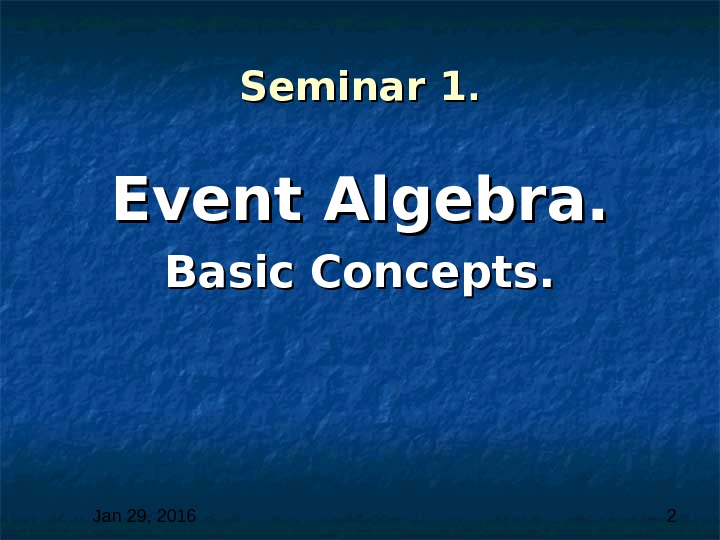Jan 29, 2016 2 Seminar 1. . Event Algebra. Basic Concepts.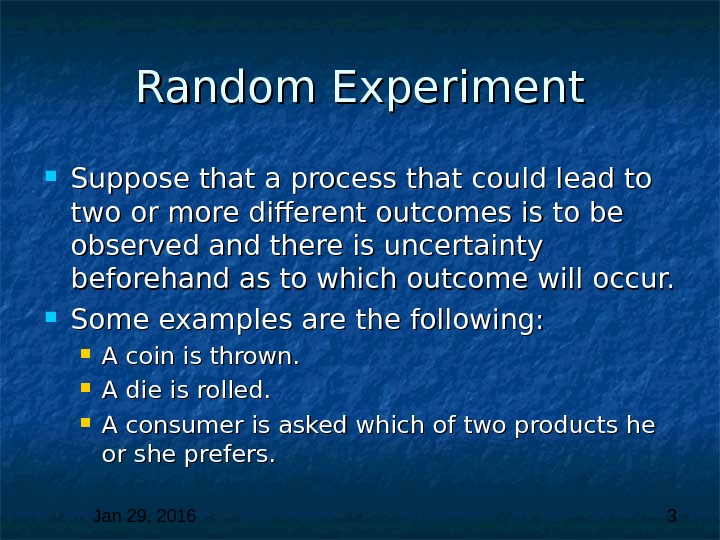Jan 29, 2016 3 Random Experiment Suppose that a process that could lead to two or more different outcomes is to be observed and there is uncertainty beforehand as to which outcome will occur. Some examples are the following: A coin is thrown. A die is rolled. A consumer is asked which of two products he or she prefers.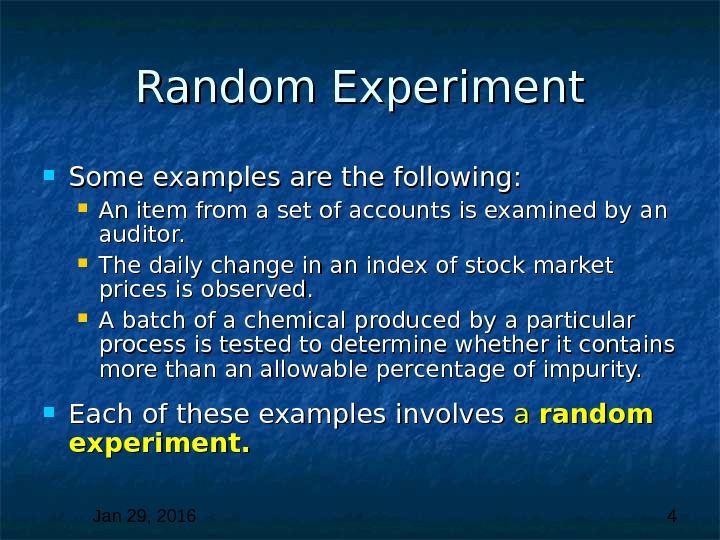Jan 29, 2016 4 Random Experiment Some examples are the following: An item from a set of accounts is examined by an auditor. The daily change in an index of stock market prices is observed. A batch of a chemical produced by a particular process is tested to determine whether it contains more than an allowable percentage of impurity. Each of these examples involves a a random experiment.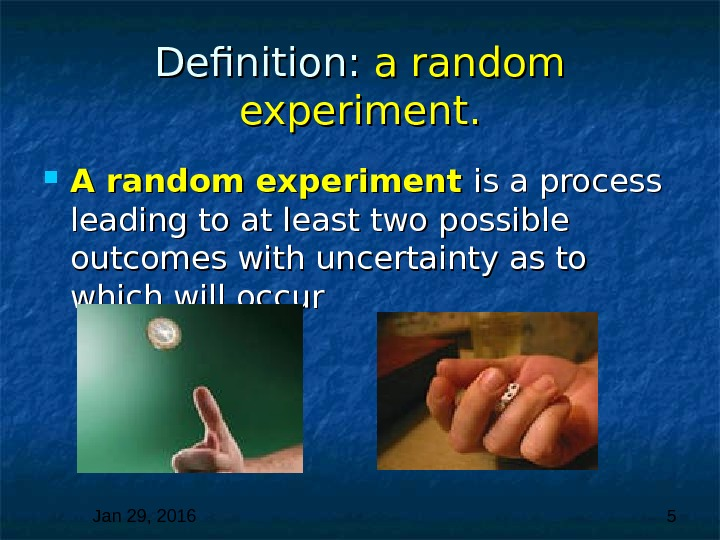Jan 29, 2016 5 Definition: a random experiment. A random experiment is a process leading to at least two possible outcomes with uncertainty as to which will occur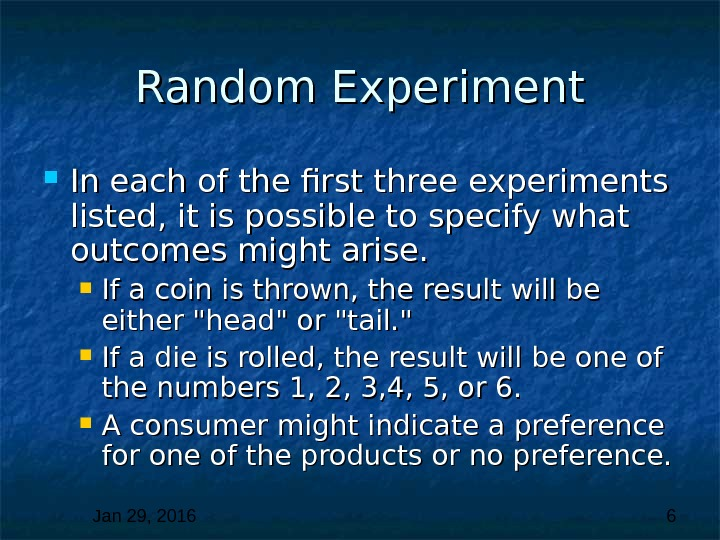Jan 29, 2016 6 Random Experiment In each of the first three experiments listed, it is possible to specify what outcomes might arise. If a coin is thrown, the result will be either «head» or «tail. » If a die is rolled, the result will be one of the numbers 1, 2, 3, 4, 5, or 6. A consumer might indicate a preference for one of the products or no preference.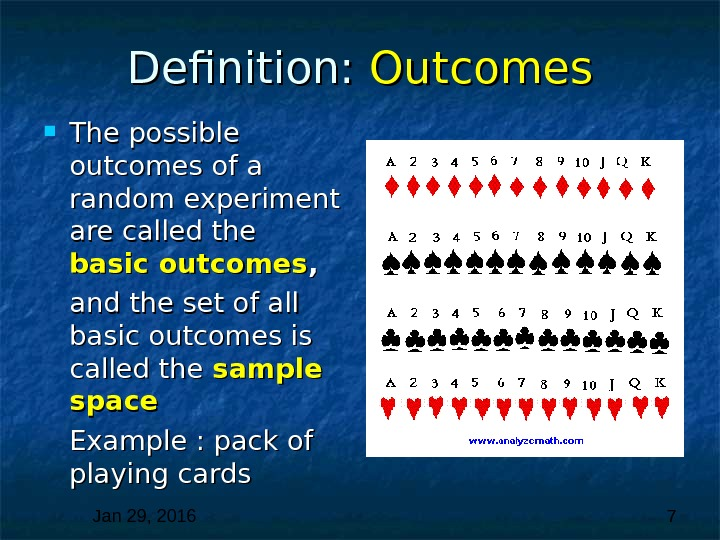Jan 29, 2016 7 Definition: Outcomes The possible outcomes of a random experiment are called the basic outcomes , , and the set of all basic outcomes is called the sample space Example : pack of playing cards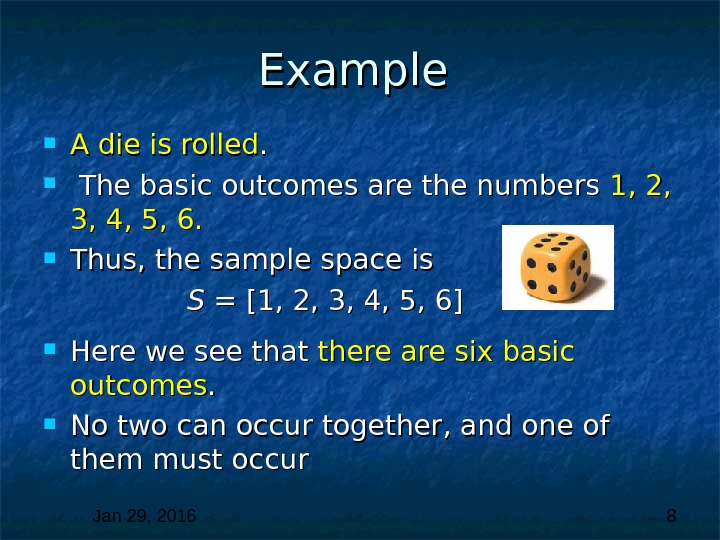Jan 29, 2016 8 Example A die is rolled. . The basic outcomes are the numbers 1, 2, 3, 4, 5, 6. Thus, the sample space is S = [1, 2, 3, 4, 5, 6] Here we see that there are six basic outcomes. . No two can occur together, and one of them must occur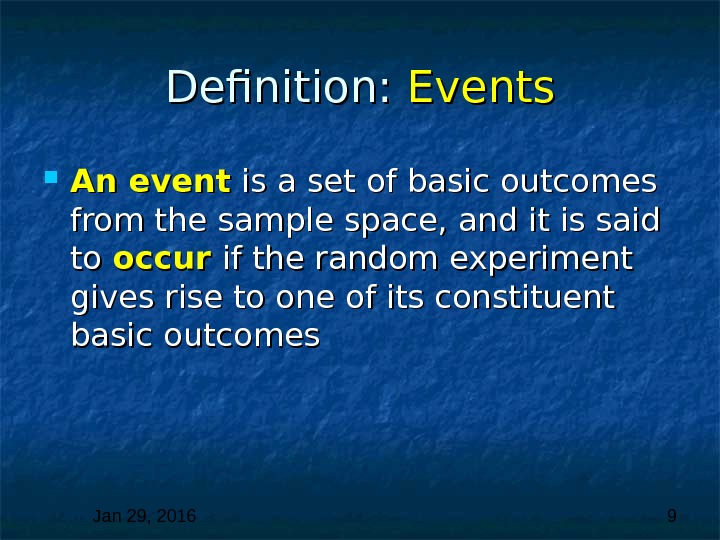Jan 29, 2016 9 Definition: Events An event is a set of basic outcomes from the sample space, and it is said to to occur if the random experiment gives rise to one of its constituent basic outcomes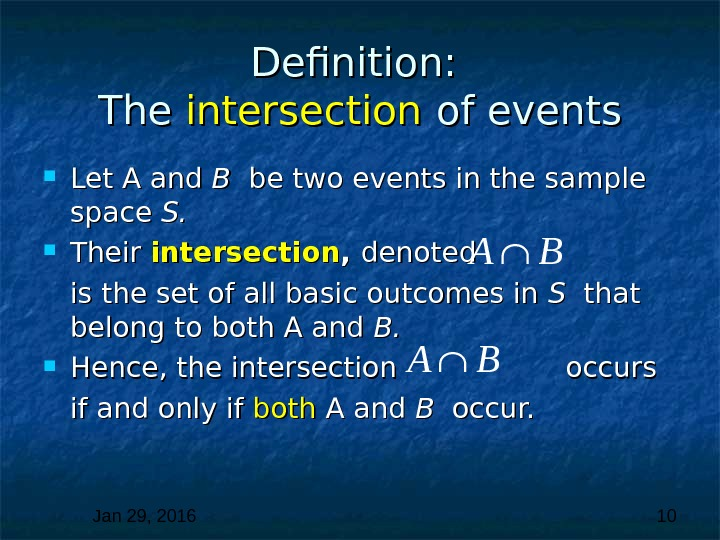Jan 29, 2016 10 Definition: The intersection of events Let A and B B be two events in the sample space S. S. Their intersection , , denoted is the set of all basic outcomes in S S that belong to both A and B. B. Hence, the intersection occurs if and only if both A and B B occur.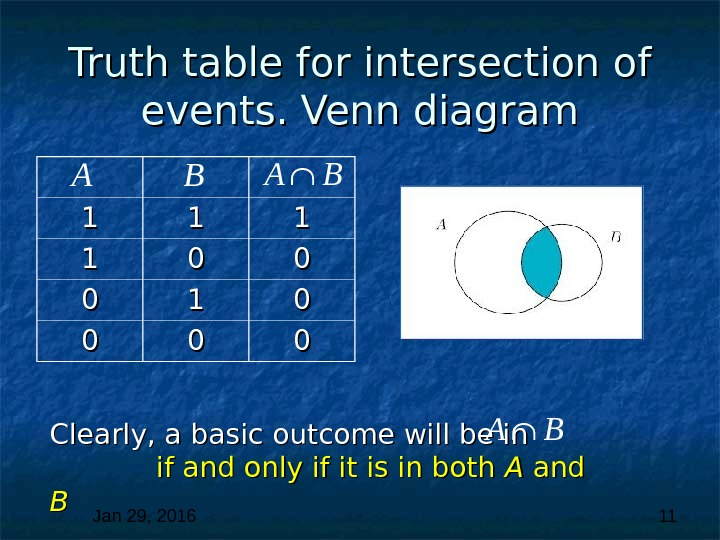Jan 29, 2016 11 Truth table for intersection of events. Venn diagram 11 11 00 00 00 11 00 00 AA BB Clearly, a basic outcome will be in if and only if it is in both A A and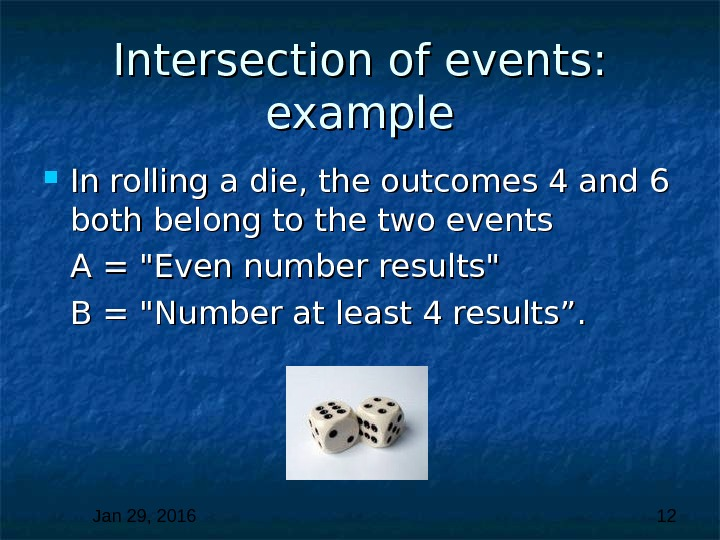Jan 29, 2016 12 Intersection of events: example In rolling a die, the outcomes 4 and 6 both belong to the two events A = «Even number results» B = «Number at least 4 results”.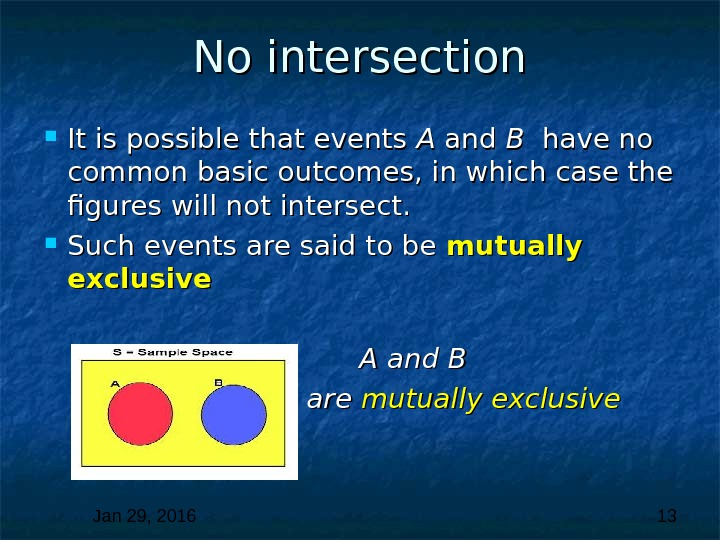Jan 29, 2016 13 No intersection It is possible that events A A and B B have no common basic outcomes, in which case the figures will not intersect. Such events are said to be mutually exclusive A and B are mutually exclusive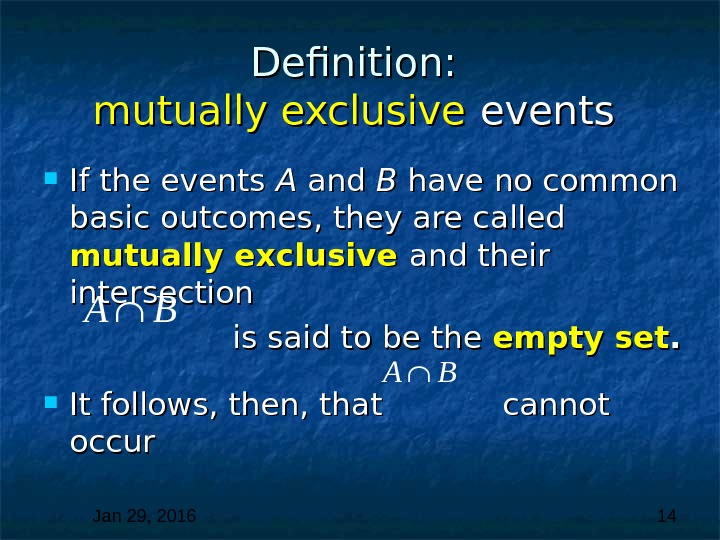Jan 29, 2016 14 Definition: mutually exclusive events If the events A A and B B have no common basic outcomes, they are called mutually exclusive and their intersection is said to be the empty set. . It follows, then, that cannot occur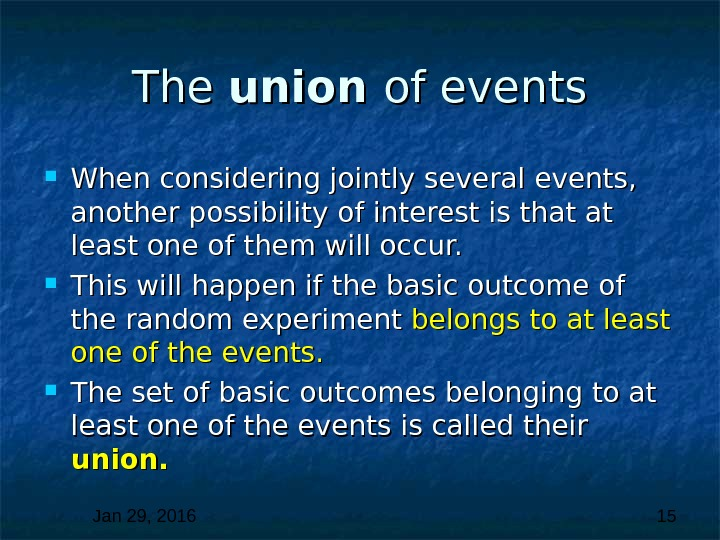Jan 29, 2016 15 The union of events When considering jointly several events, another possibility of interest is that at least one of them will occur. This will happen if the basic outcome of the random experiment belongs to at least one of the events. The set of basic outcomes belonging to at least one of the events is called their union.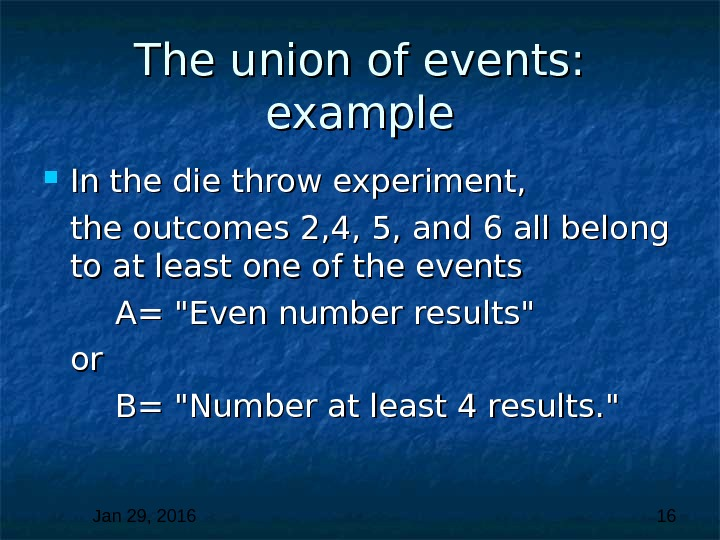Jan 29, 2016 16 The union of events: example In the die throw experiment, the outcomes 2, 4, 5, and 6 all belong to at least one of the events A= «Even number results» or or B= «Number at least 4 results. «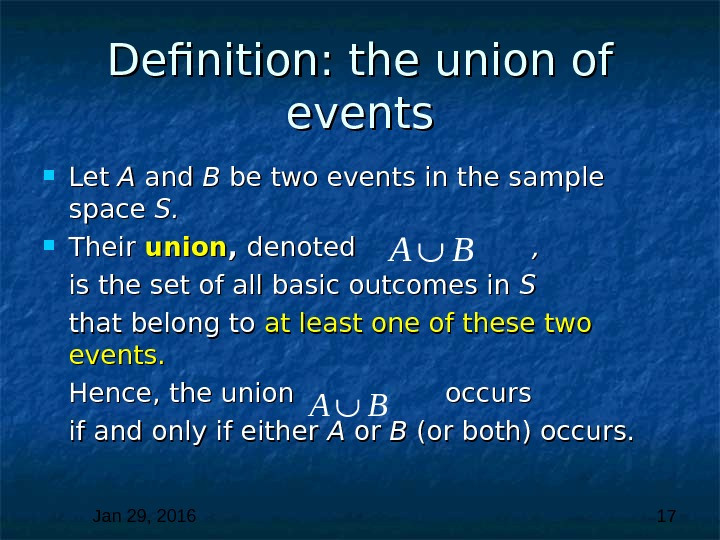Jan 29, 2016 17 Definition: the union of events Let A A and B B be two events in the sample space S. S. Their union , , denoted , is the set of all basic outcomes in S S that belong to at least one of these two events. Hence, the union occurs if and only if either A A or or B B (or both) occurs.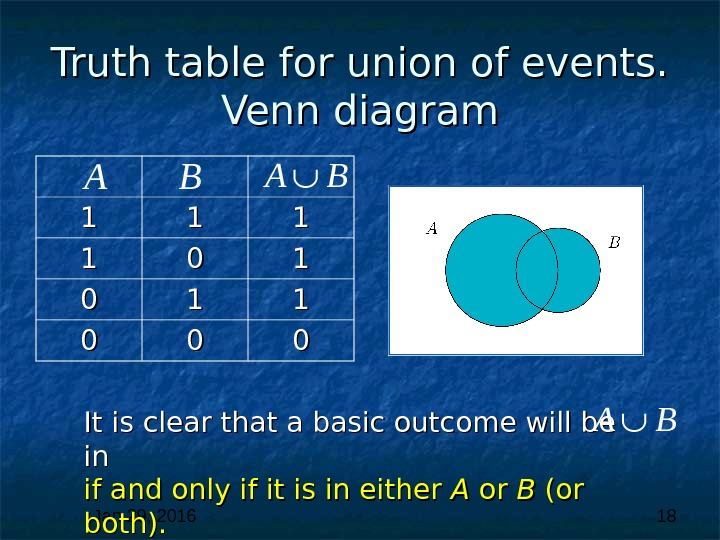Jan 29, 2016 18 Truth table for union of events. Venn diagram 11 11 00 00 00 A B It is clear that a basic outcome will be in in if and only if it is in either A A or or B B (or both).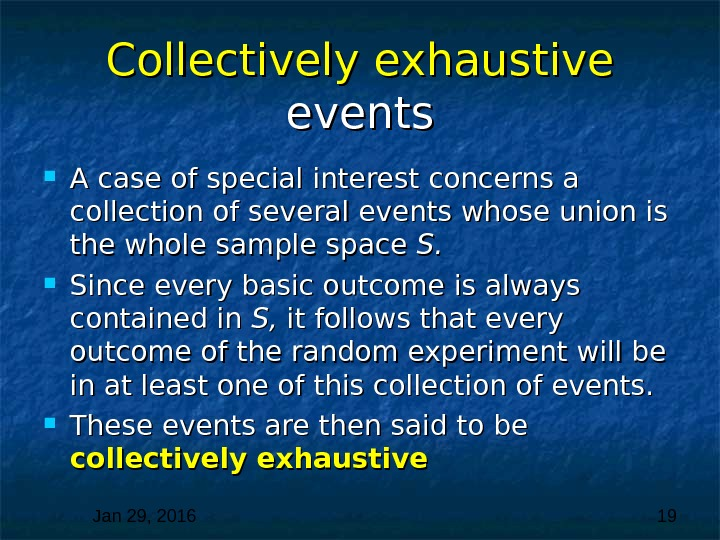Jan 29, 2016 19 Collectively exhaustive events A case of special interest concerns a collection of several events whose union is the whole sample space S. S. Since every basic outcome is always contained in S, S, it follows that every outcome of the random experiment will be in at least one of this collection of events. These events are then said to be collectively exhaustive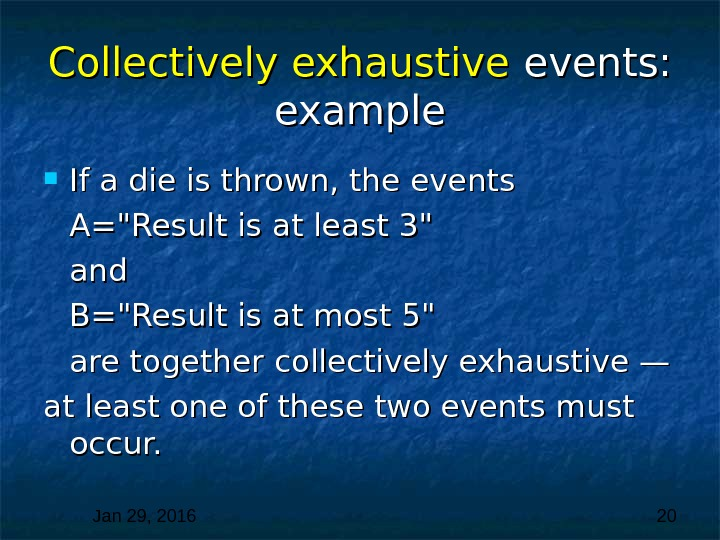Jan 29, 2016 20 Collectively exhaustive events: example If a die is thrown, the events A=»Result is at least 3″ and B=»Result is at most 5″ are together collectively exhaustive — at least one of these two events must occur.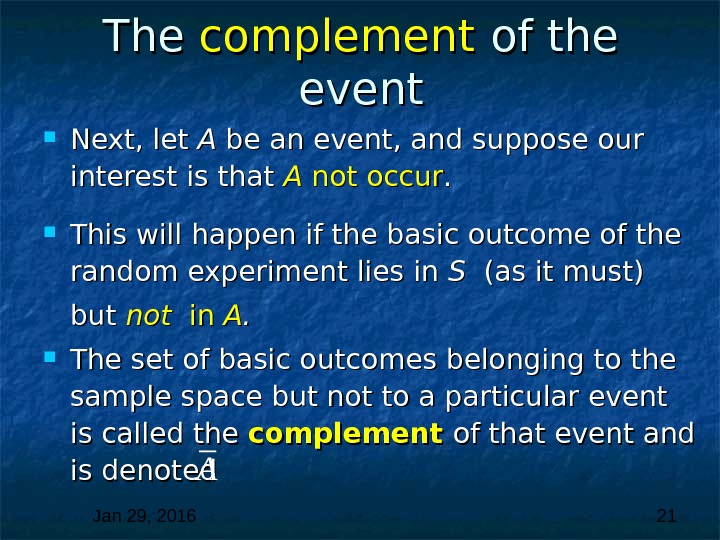Jan 29, 2016 21 The complement of the event Next, let A A be an event, and suppose our interest is that A A not occur. . This will happen if the basic outcome of the random experiment lies in S S (as it must) but not in in AA. . The set of basic outcomes belonging to the sample space but not to a particular event is called the complement of that event and is denoted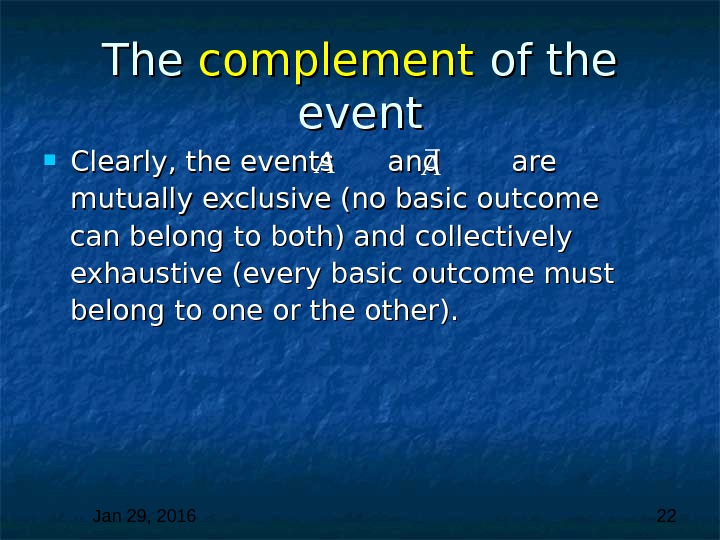Jan 29, 2016 22 The complement of the event Clearly, the events and are mutually exclusive (no basic outcome can belong to both) and collectively exhaustive (every basic outcome must belong to one or the other).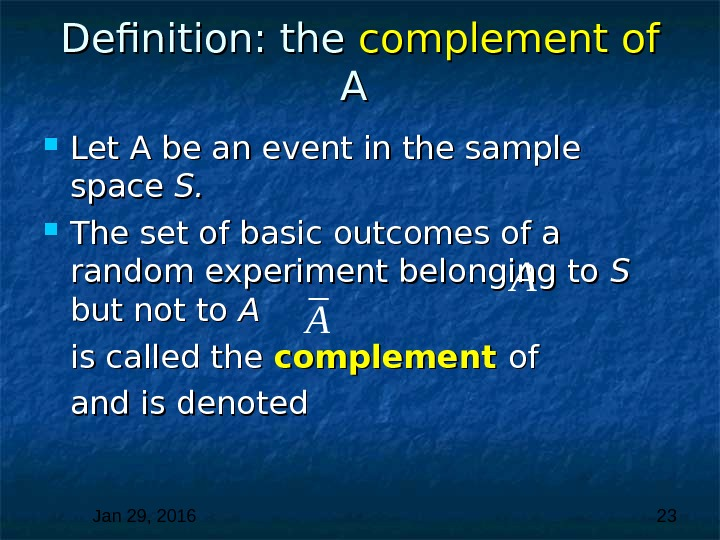Jan 29, 2016 23 Definition: the complement of A A Let A be an event in the sample space S. S. The set of basic outcomes of a random experiment belonging to S S but not to A A is called the complement ofof and is denoted.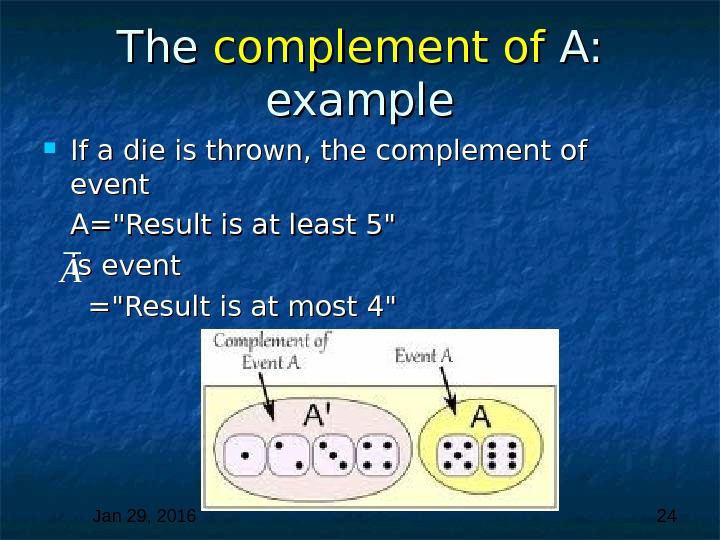Jan 29, 2016 24 The complement of A: example If a die is thrown, the complement of event A=»Result is at least 5″ is event =»Result is at most 4″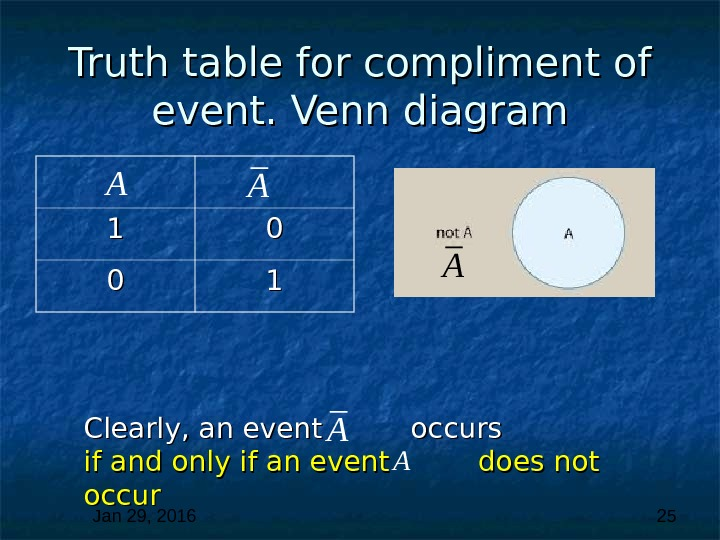Jan 29, 2016 25 Truth table for compliment of event. Venn diagram 11 00 00 11 AA Clearly, an event occurs if and only if an event does not occur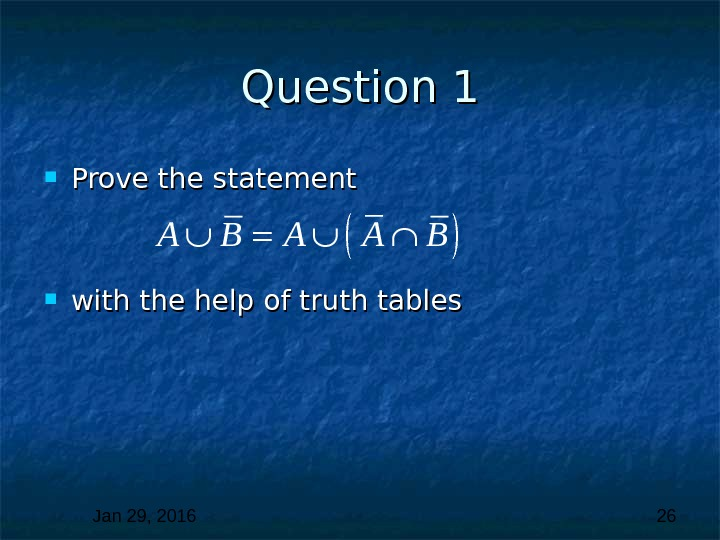Jan 29, 2016 26 Question 1 1 Prove the statement with the help of truth tables.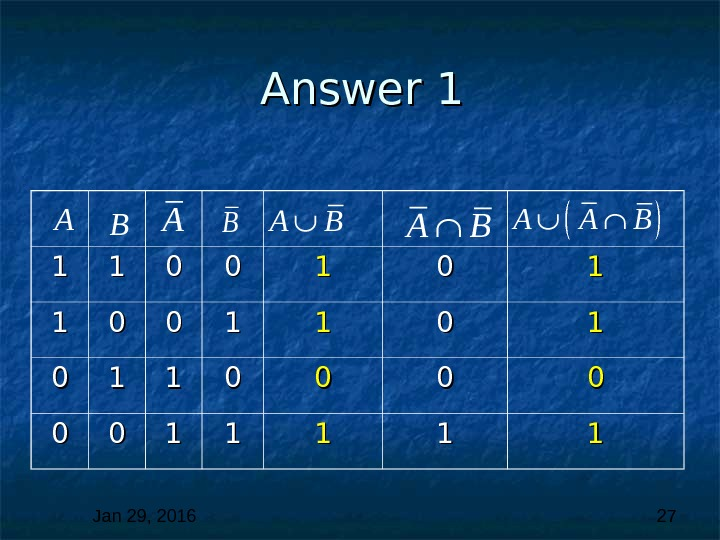Jan 29, 2016 27 Answer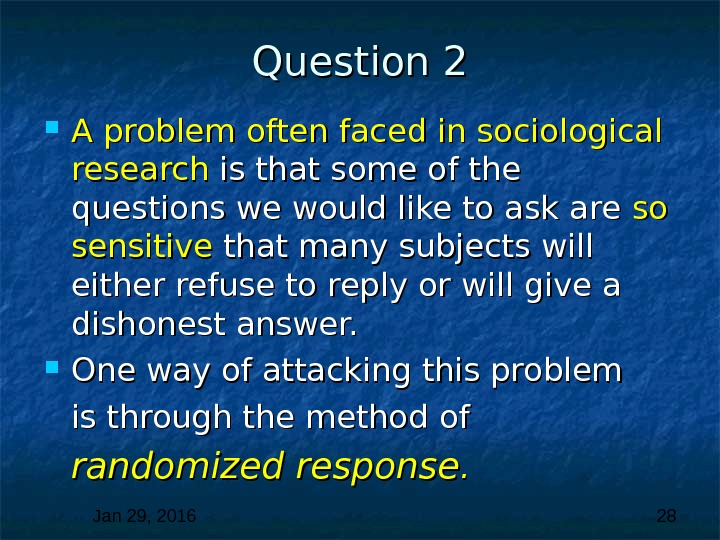Jan 29, 2016 28 Question 2 A problem often faced in sociological research is that some of the questions we would like to ask are so so sensitive that many subjects will either refuse to reply or will give a dishonest answer. One way of attacking this problem is through the method of randomized response. .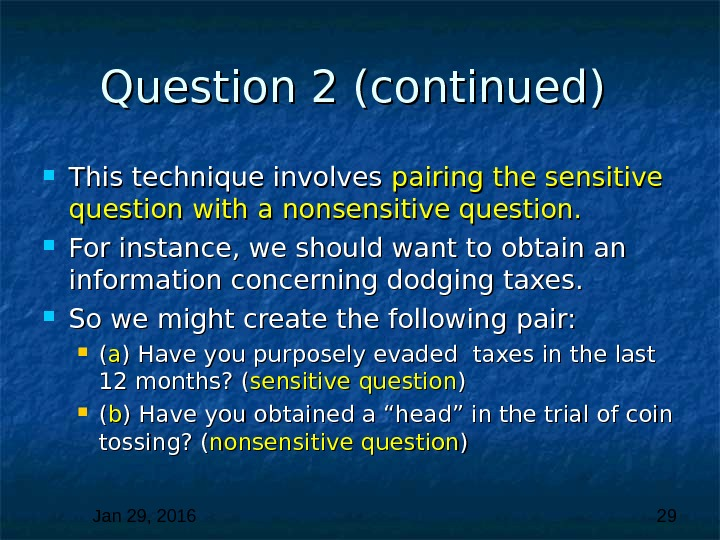Jan 29, 2016 29 Question 2 (continued) This technique involves pairing the sensitive question with a nonsensitive question. For instance, we should want to obtain an information concerning dodging taxes. So we might create the following pair: (( aa ) Have you purposely evaded taxes in the last 12 months? ( sensitive question )) (( bb ) Have you obtained a “head” in the trial of coin tossing? ( nonsensitive question ))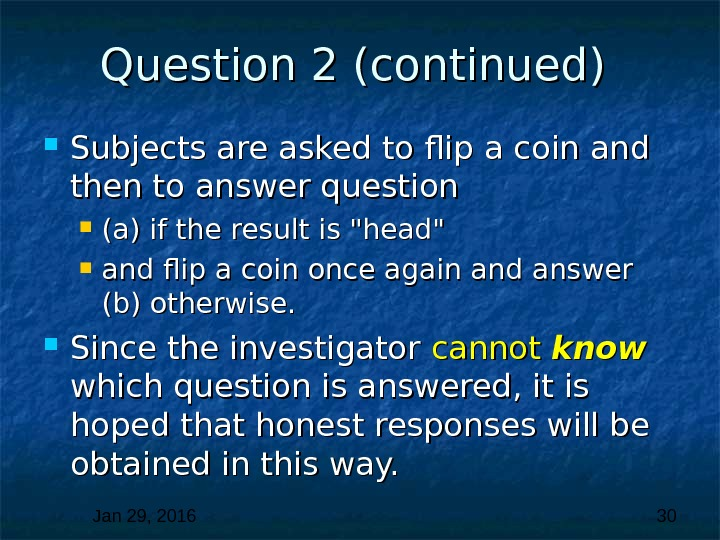Jan 29, 2016 30 Question 2 (continued) Subjects are asked to flip a coin and then to answer question (a) if the result is «head» and flip a coin once again and answer (b) otherwise. Since the investigator cannot know which question is answered, it is hoped that honest responses will be obtained in this way.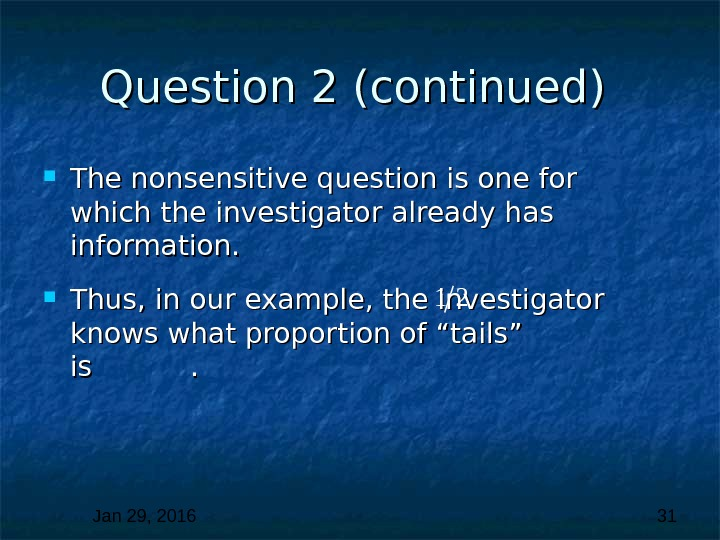Jan 29, 2016 31 Question 2 (continued) The nonsensitive question is one for which the investigator already has information. Thus, in our example, the investigator knows what proportion of “tails” is .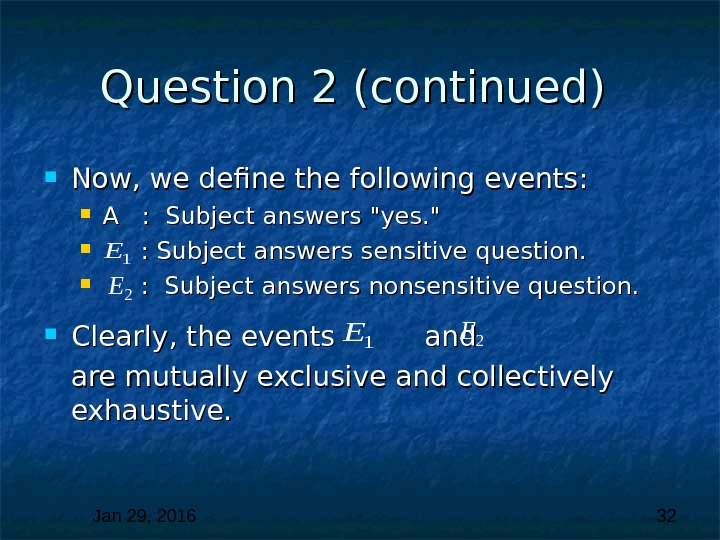Jan 29, 2016 32 Question 2 (continued) Now, we define the following events: A : Subject answers «yes. » : Subject answers sensitive question. : : Subject answers nonsensitive question. Clearly, the events and are mutually exclusive and collectively exhaustive.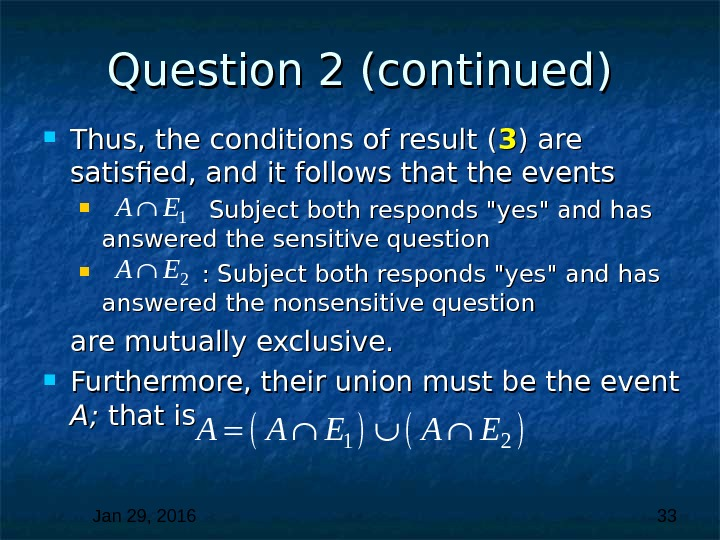Jan 29, 2016 33 Question 2 (continued) Thus, the conditions of result ( 33 ) are satisfied, and it follows that the events Subject both responds «yes» and has answered the sensitive question : : Subject both responds «yes» and has answered the nonsensitive question are mutually exclusive. Furthermore, their union must be the event A; A; that is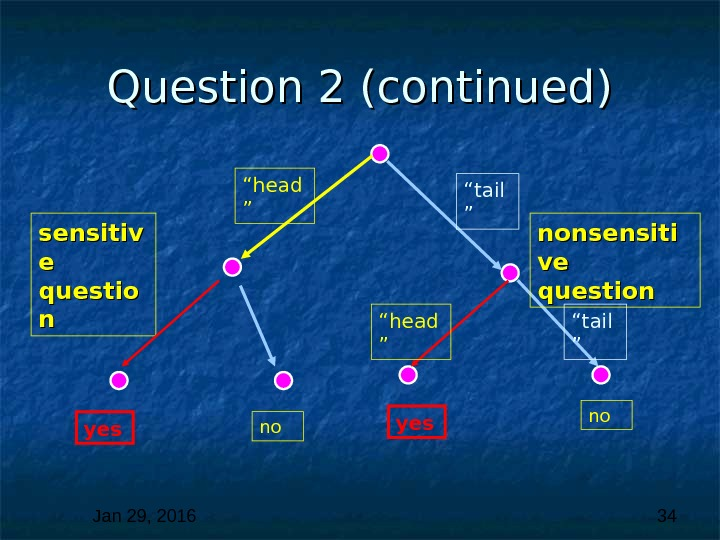Jan 29, 2016 34 Question 2 (continued) “ head ” “ tail ” sensitiv e e questio nn yes nononsensiti ve ve question “ head ” “ tail ”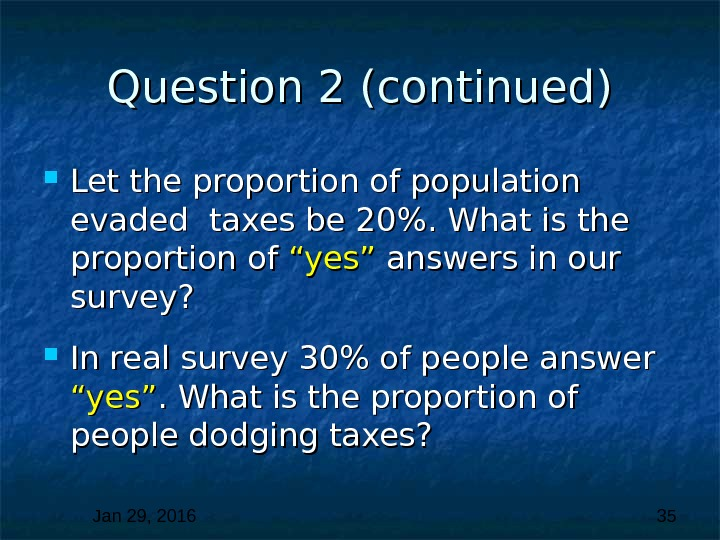Jan 29, 2016 35 Question 2 (continued) Let the proportion of population evaded taxes be 20%. What is the proportion of “yes” answers in our survey? In real survey 30% of people answer “yes”. What is the proportion of people dodging taxes?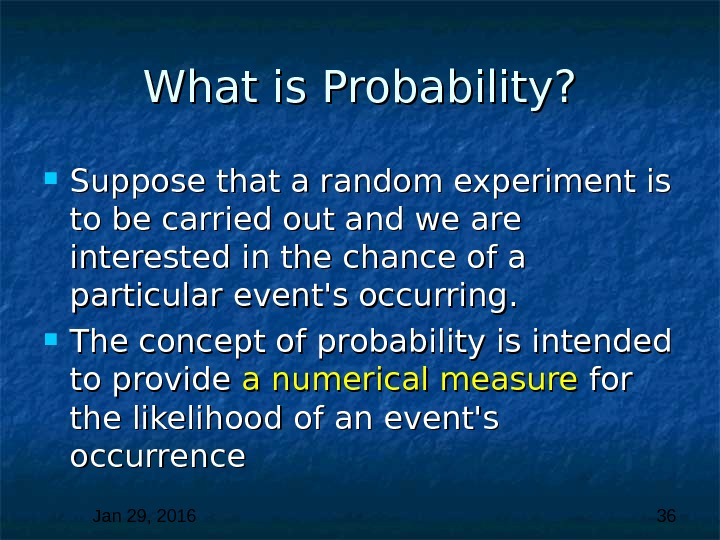Jan 29, 2016 36 What is Probability? Suppose that a random experiment is to be carried out and we are interested in the chance of a particular event’s occurring. The concept of probability is intended to provide a numerical measure for the likelihood of an event’s occurrence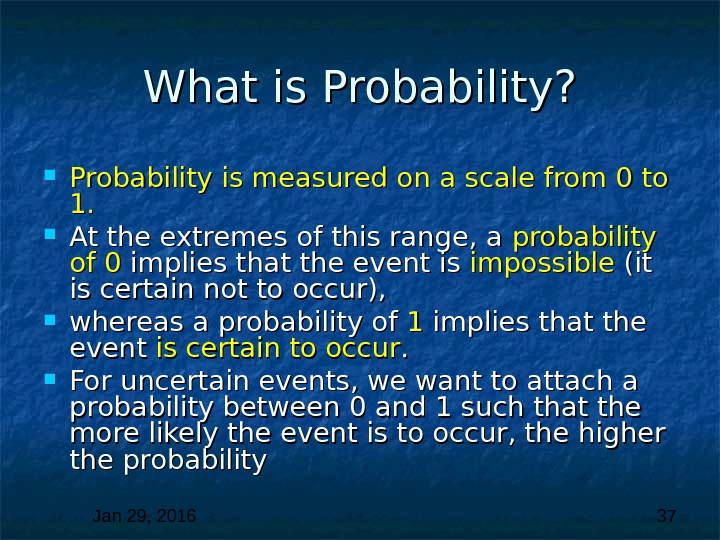Jan 29, 2016 37 What is Probability? Probability is measured on a scale from 0 to 1. 1. At the extremes of this range, a probability ofof 0 0 implies that the event is impossible (it is certain not to occur), whereas a probability of 11 implies that the event is certain to occur. . For uncertain events, we want to attach a probability between 0 and 1 such that the more likely the event is to occur, the higher the probability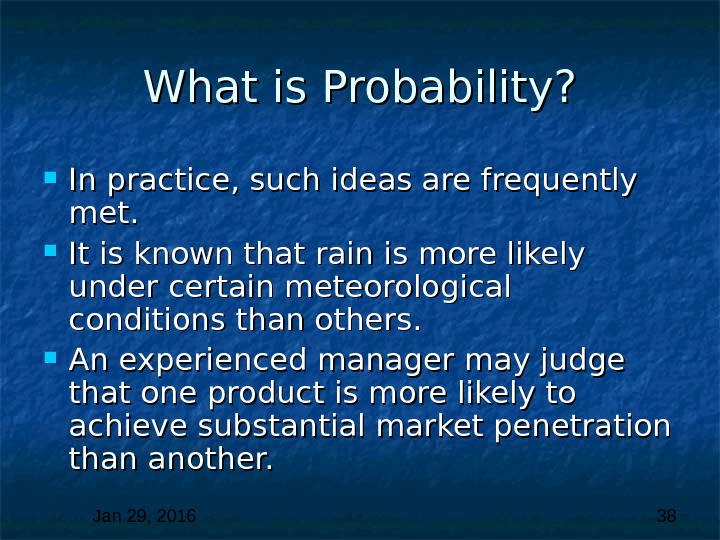Jan 29, 2016 38 What is Probability? In practice, such ideas are frequently met. It is known that rain is more likely under certain meteorological conditions than others. An experienced manager may judge that one product is more likely to achieve substantial market penetration than another.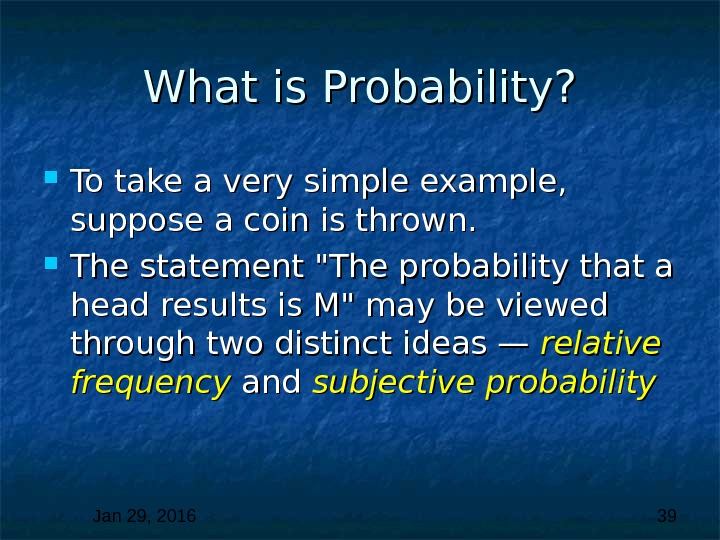Jan 29, 2016 39 What is Probability? To take a very simple example, suppose a coin is thrown. The statement «The probability that a head results is M» may be viewed through two distinct ideas — relative frequency and subjective probability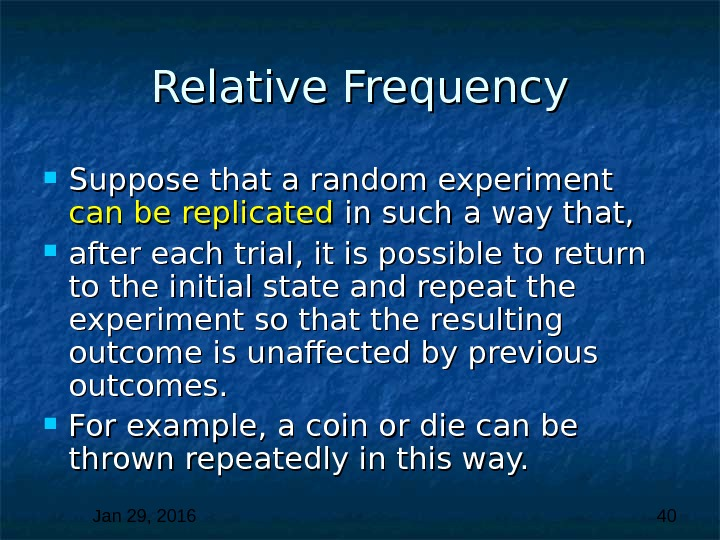Jan 29, 2016 40 Relative Frequency Suppose that a random experiment can be replicated in such a way that, after each trial, it is possible to return to the initial state and repeat the experiment so that the resulting outcome is unaffected by previous outcomes. For example, a coin or die can be thrown repeatedly in this way.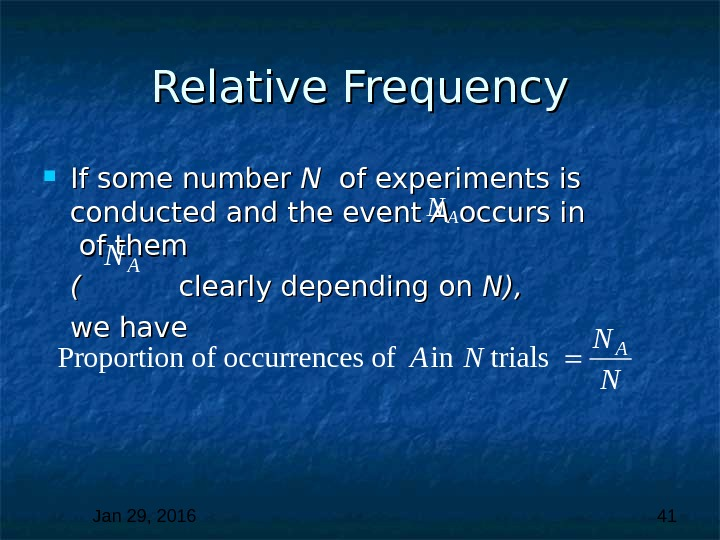Jan 29, 2016 41 Relative Frequency If some number N N of experiments is conducted and the event A A occurs in of them ( ( clearly depending on N), we have AN A N Proportion of occurrences of in trials A N N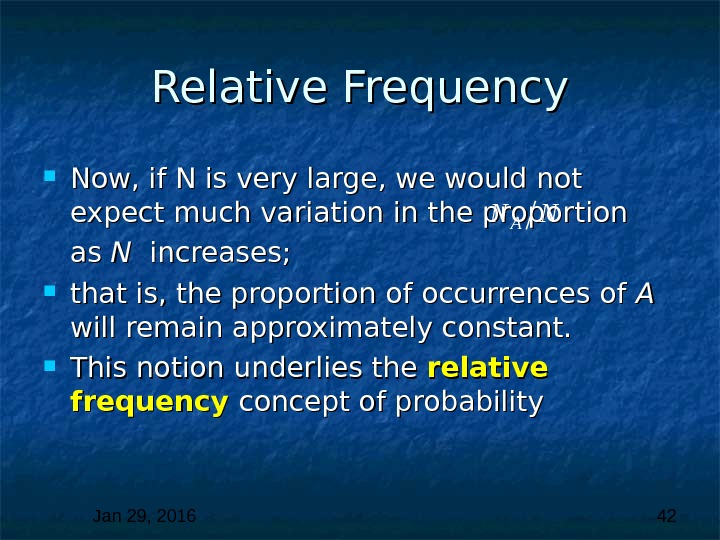Jan 29, 2016 42 Relative Frequency Now, if N is very large, we would not expect much variation in the proportion as as N N increases; that is, the proportion of occurrences of A A will remain approximately constant. This notion underlies the relative frequency concept of probability AN N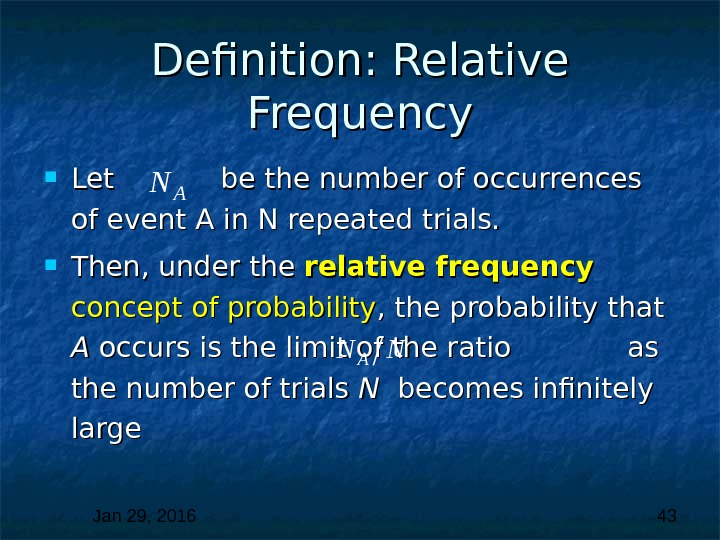Jan 29, 2016 43 Definition: Relative Frequency Let be the number of occurrences of event A in N repeated trials. Then, under the relative frequency concept of probability , the probability that A A occurs is the limit of the ratio as as the number of trials N N becomes infinitely large AN AN N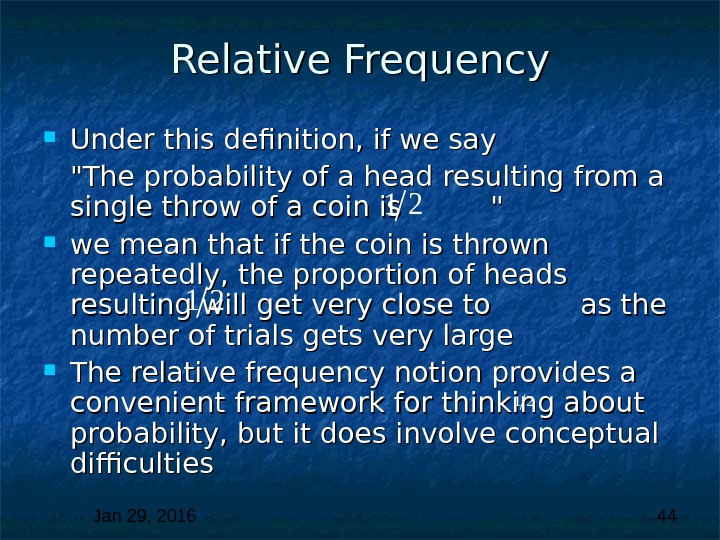Jan 29, 2016 44 Relative Frequency Under this definition, if we say «The probability of a head resulting from a single throw of a coin is » » we mean that if the coin is thrown repeatedly, the proportion of heads resulting will get very close to as the number of trials gets very large The relative frequency notion provides a convenient framework for thinking about probability, but it does involve conceptual difficulties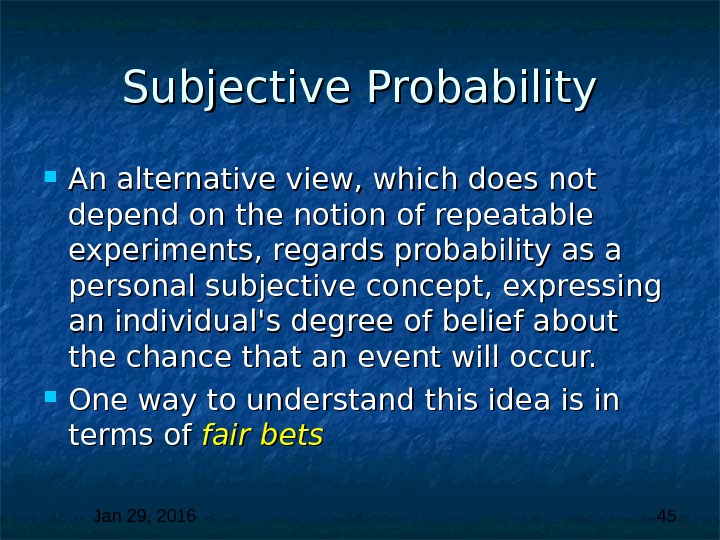Jan 29, 2016 45 Subjective Probability An alternative view, which does not depend on the notion of repeatable experiments, regards probability as a personal subjective concept, expressing an individual’s degree of belief about the chance that an event will occur. One way to understand this idea is in terms of fair bets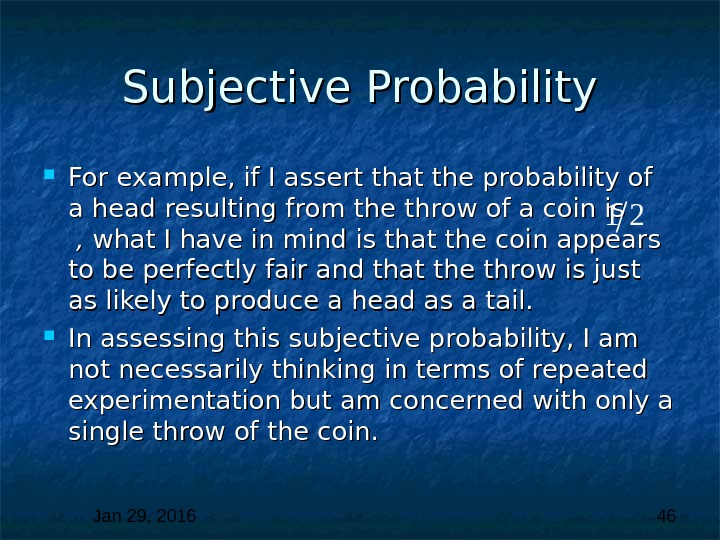Jan 29, 2016 46 Subjective Probability For example, if I assert that the probability of a head resulting from the throw of a coin is , , what I have in mind is that the coin appears to be perfectly fair and that the throw is just as likely to produce a head as a tail. In assessing this subjective probability, I am not necessarily thinking in terms of repeated experimentation but am concerned with only a single throw of the coin.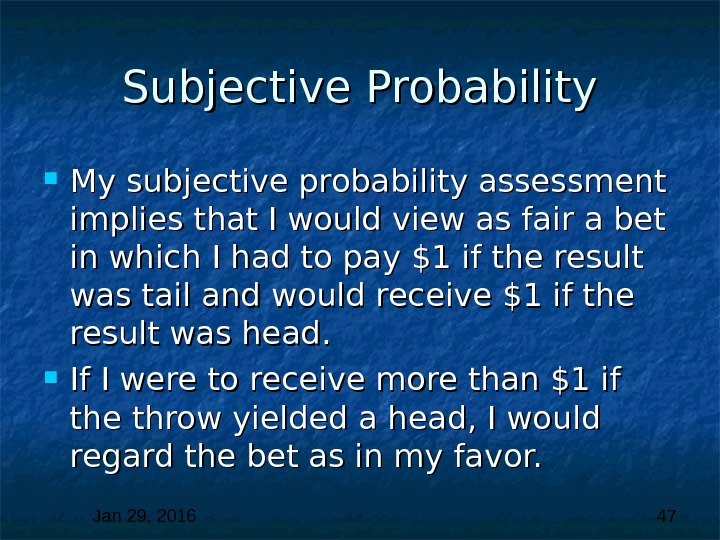Jan 29, 2016 47 Subjective Probability My subjective probability assessment implies that I would view as fair a bet in which I had to pay \$1 if the result was tail and would receive \$1 if the result was head. If I were to receive more than \$1 if the throw yielded a head, I would regard the bet as in my favor.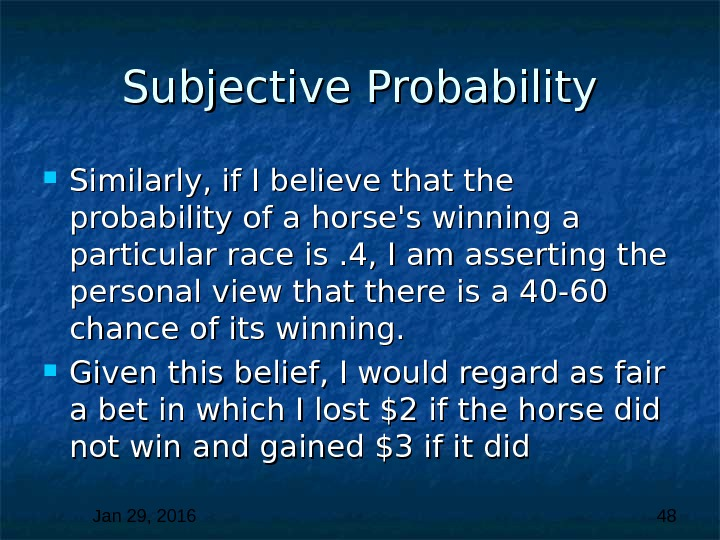Jan 29, 2016 48 Subjective Probability Similarly, if I believe that the probability of a horse’s winning a particular race is. 4, I am asserting the personal view that there is a 40 -60 chance of its winning. Given this belief, I would regard as fair a bet in which I lost \$2 if the horse did not win and gained \$3 if it did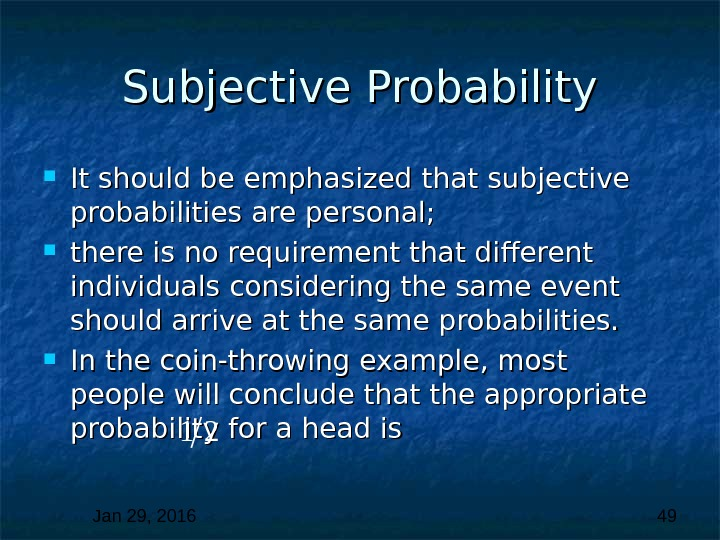Jan 29, 2016 49 Subjective Probability It should be emphasized that subjective probabilities are personal; there is no requirement that different individuals considering the same event should arrive at the same probabilities. In the coin-throwing example, most people will conclude that the appropriate probability for a head is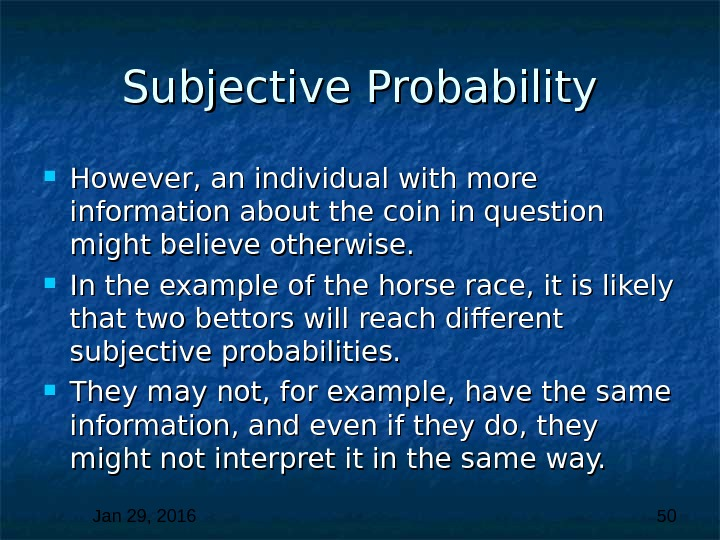Jan 29, 2016 50 Subjective Probability However, an individual with more information about the coin in question might believe otherwise. In the example of the horse race, it is likely that two bettors will reach different subjective probabilities. They may not, for example, have the same information, and even if they do, they might not interpret it in the same way.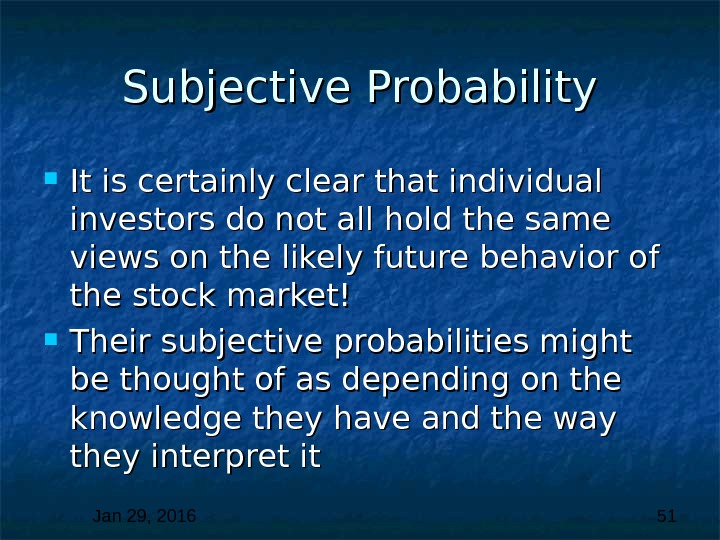Jan 29, 2016 51 Subjective Probability It is certainly clear that individual investors do not all hold the same views on the likely future behavior of the stock market! Their subjective probabilities might be thought of as depending on the knowledge they have and the way they interpret it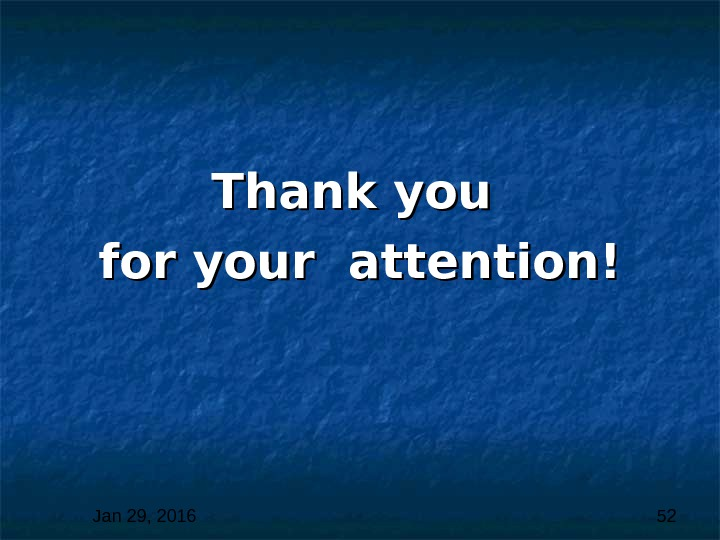Jan 29, 2016 52 Thank you for your attention!